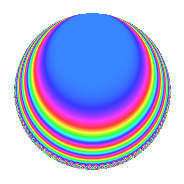# Properties

 Label 4032.2.b.kLevel 4032 Weight 2 Character orbit 4032.b Analytic conductor 32.196 Analytic rank 0 Dimension 4 CM disc. -84 Inner twists 8

# Related objects

## Newspace parameters

 Level: $$N$$ = $$4032 = 2^{6} \cdot 3^{2} \cdot 7$$ Weight: $$k$$ = $$2$$ Character orbit: $$[\chi]$$ = 4032.b (of order $$2$$ and degree $$1$$)

## Newform invariants

 Self dual: No Analytic conductor: $$32.195682095$$ Analytic rank: $$0$$ Dimension: $$4$$ Coefficient field: $$\Q(\sqrt{-2}, \sqrt{7})$$ Coefficient ring: $$\Z[a_1, \ldots, a_{11}]$$ Coefficient ring index: $$2$$ Sato-Tate group: $\mathrm{U}(1)[D_{2}]$

## $q$-expansion

Coefficients of the $$q$$-expansion are expressed in terms of a basis $$1,\beta_1,\beta_2,\beta_3$$ for the coefficient ring described below. We also show the integral $$q$$-expansion of the trace form.

 $$f(q)$$ $$=$$ $$q + \beta_{2} q^{5} -\beta_{3} q^{7} +O(q^{10})$$ $$q + \beta_{2} q^{5} -\beta_{3} q^{7} -\beta_{1} q^{11} -\beta_{2} q^{17} + 2 \beta_{3} q^{19} -5 \beta_{1} q^{23} -9 q^{25} + 4 \beta_{3} q^{31} -7 \beta_{1} q^{35} -8 q^{37} -\beta_{2} q^{41} + 7 q^{49} + 2 \beta_{3} q^{55} -11 \beta_{1} q^{71} + \beta_{2} q^{77} + 14 q^{85} + 5 \beta_{2} q^{89} + 14 \beta_{1} q^{95} +O(q^{100})$$ $$\operatorname{Tr}(f)(q)$$ $$=$$ $$4q + O(q^{10})$$ $$4q - 36q^{25} - 32q^{37} + 28q^{49} + 56q^{85} + O(q^{100})$$

Basis of coefficient ring in terms of a root $$\nu$$ of $$x^{4} + 8 x^{2} + 9$$:

 $$\beta_{0}$$ $$=$$ $$1$$ $$\beta_{1}$$ $$=$$ $$($$$$\nu^{3} + 5 \nu$$$$)/3$$ $$\beta_{2}$$ $$=$$ $$($$$$\nu^{3} + 11 \nu$$$$)/3$$ $$\beta_{3}$$ $$=$$ $$\nu^{2} + 4$$
 $$1$$ $$=$$ $$\beta_0$$ $$\nu$$ $$=$$ $$($$$$\beta_{2} - \beta_{1}$$$$)/2$$ $$\nu^{2}$$ $$=$$ $$\beta_{3} - 4$$ $$\nu^{3}$$ $$=$$ $$($$$$-5 \beta_{2} + 11 \beta_{1}$$$$)/2$$

## Character Values

We give the values of $$\chi$$ on generators for $$\left(\mathbb{Z}/4032\mathbb{Z}\right)^\times$$.

 $$n$$ $$127$$ $$577$$ $$1793$$ $$3781$$ $$\chi(n)$$ $$-1$$ $$-1$$ $$1$$ $$1$$

## Embeddings

For each embedding $$\iota_m$$ of the coefficient field, the values $$\iota_m(a_n)$$ are shown below.

For more information on an embedded modular form you can click on its label.

Label $$\iota_m(\nu)$$ $$a_{2}$$ $$a_{3}$$ $$a_{4}$$ $$a_{5}$$ $$a_{6}$$ $$a_{7}$$ $$a_{8}$$ $$a_{9}$$ $$a_{10}$$
3583.1
 − 1.16372i − 2.57794i 1.16372i 2.57794i
0 0 0 3.74166i 0 −2.64575 0 0 0
3583.2 0 0 0 3.74166i 0 2.64575 0 0 0
3583.3 0 0 0 3.74166i 0 −2.64575 0 0 0
3583.4 0 0 0 3.74166i 0 2.64575 0 0 0
 $$n$$: e.g. 2-40 or 990-1000 Significant digits: Format: Complex embeddings Normalized embeddings Satake parameters Satake angles

## Inner twists

Char. orbit Parity Mult. Self Twist Proved
1.a Even 1 trivial yes
84.h Odd 1 CM by $$\Q(\sqrt{-21})$$ yes
3.b Odd 1 yes
4.b Odd 1 yes
7.b Odd 1 yes
12.b Even 1 yes
21.c Even 1 yes
28.d Even 1 yes

## Hecke kernels

This newform can be constructed as the intersection of the kernels of the following linear operators acting on $$S_{2}^{\mathrm{new}}(4032, [\chi])$$:

 $$T_{5}^{2} + 14$$ $$T_{11}^{2} + 2$$ $$T_{19}^{2} - 28$$